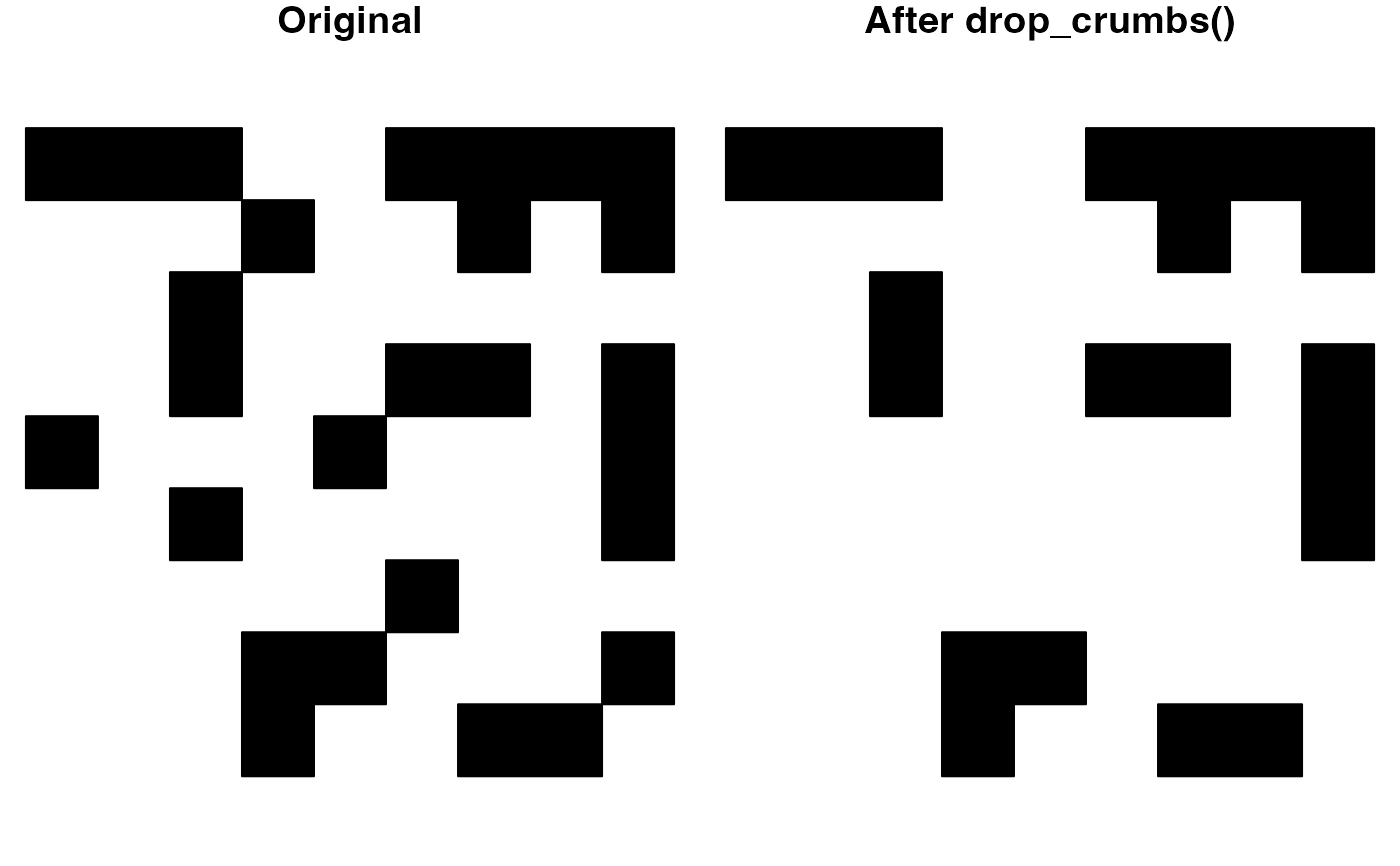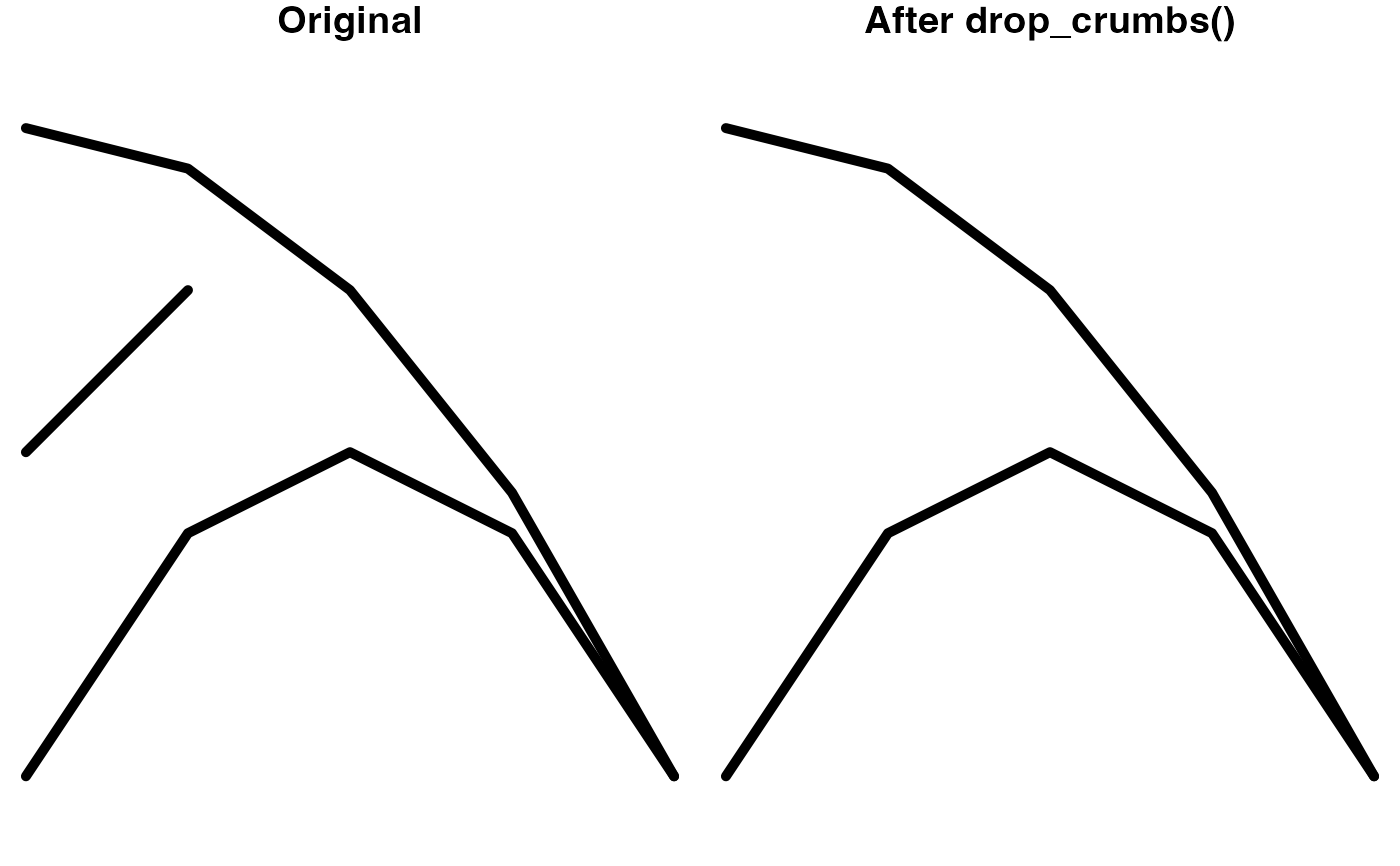Remove polygons or line segments below a given area or length threshold.

drop_crumbs(x, threshold, drop_empty = TRUE)

## Arguments

x spatial features; lines or polygons from either the sf, sp, or terra packages. an area or length threshold, below which features will be removed. Provided either as a units object (see units::set_units()), or a numeric threshold in the units of the coordinate reference system. If x is in unprojected coordinates, a numeric threshold is assumed to be in meters. logical; whether features with sizes below the given threshold should be removed (the default) or kept as empty geometries. Note that sp objects cannot store empty geometries, so this argument will be ignored and empty geometries will always be removed.

## Value

A spatial feature, with small pieces removed, in the same format as the input data. If none of the features are larger than the threshold, sf inputs will return a geometry set with zero features, and sp inputs will return NULL.

## Details

For multipart features, the removal threshold is applied to the individual components. This means that, in some cases, an entire feature may be removed, while in other cases, only parts of the multipart feature will be removed.

## Examples

# remove polygons smaller than 200km2
p <- jagged_polygons$geometry area_thresh <- units::set_units(200, km^2) p_dropped <- drop_crumbs(p, threshold = area_thresh) # plot par(mar = c(0, 0, 1, 0), mfrow = c(1, 2)) plot(p, col = "black", main = "Original") if (length(p_dropped) > 0) { plot(p_dropped, col = "black", main = "After drop_crumbs()") }# remove lines less than 25 miles l <- jagged_lines$geometry
# note that any units can be used
# conversion to units of projection happens automatically
length_thresh <- units::set_units(25, miles)
l_dropped <- drop_crumbs(l, threshold = length_thresh)
# plot
par(mar = c(0, 0, 1, 0), mfrow = c(1, 2))
plot(l, lwd = 5, main = "Original")
if (length(l_dropped)) {
plot(l_dropped, lwd = 5, main = "After drop_crumbs()")
}